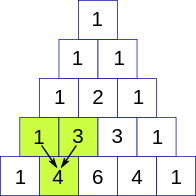New update is available. Click here to update.
Last Updated: Apr 16, 2023

# Count all binary strings having length N and contain at least X 0's and Y 1's.Author Vaibhav Agarwal0 upvotes

## Introduction

### Problem Statement

The problem states that we are given three integers, i.e., N, X, Y. We need to find the count of all possible binary strings having length N and contain at least X 0’s and Y 1’s.

Let’s discuss the sample test case. It will be more clear:  :

### Sample Examples

Example 1:

``````Input:
N = 3, X = 1, Y = 1

Output:
6

Explanation:
All possible binary strings having length 3 and containing at least 1 0’s and 1 1’s are “001”, “010”, “100”, “011”, “101”, “110”. ``````

Example 2:

``````Input:
N = 5, X = 2, Y = 2

Output:
20``````

## Naive Approach

In the brute force approach, the idea is to generate all binary strings of length N and then check each generated binary string for at least X 0’s and Y 1’s. If a string satisfies the condition, increase the count and return the final count.

Time ComplexityO(2^N)

Explanation: There are 2^N possible binary strings of length N.

Space ComplexityO(1)

Explanation: No extra space is required.

## Optimized Approach(Combinatorics)

The idea is to use combinatorics. If the length of the string is N, and suppose it contains X 0’s, then there will be Y = (N-X) 1’s. To find out the number of distinct binary strings containing X 0’s, it will be equal to NCX. Now to find all possible binary strings that contain at least X 0’s and at least Y 1’s, we calculate  NCi for all i, where i = [X, N-Y], and it to a variable, let say sum. The value of sum after all iterations will be our required count.

Now the main task is to calculate NCr efficiently. We will use the pascal triangle to calculate the value of NCr

Pascal’s Triangle

A Pascal's triangle is a triangular array constructed by summing adjacent elements in preceding rows. Pascal's triangle contains the values of the binomial coefficient.Source - Coding Ninjas

A pascal’s triangle can be used to find the value of nCr Since the formula of  nCr = n!/(r! * (n-r)!) it is also the formula for the cell of the pascal’s triangle. So if we create pascal’s triangle, we can get the value of nCr, in the nth row and rth column.

We will create the pascal’s triable using the conditions:

1. The number of ways of selecting 0 elements from the n elements will be 1, i.e., nC0 = 1.
2. Number of ways of selecting r elements from n elements will be equal to the sum of ways of selecting r-1 elements from n-1 elements and select r elements from n-1 elements, i.e, nCr = (n-1)C(r-1) + (n-1)C(r)

Now let’s Look at the algorithms for finding the total number of binary strings having at least X 0’s and Y 1’s.

### Steps of Algorithm

1. Create the pascal’s triangle of N x N using matrix pas[][].
2. Initialize the variable sum = 0 to store the total count of ways of finding the binary strings.
3. Start traversing from [X, N-Y] using for loop.
4. In each iteration, find the value of pas[N][i], X<=i<=N-Y, and add to the variable sum.
5. The calculated sum variable will contain the total number of binary strings with at least X 0’s and Y 1’s.

### Implementation in C++

``````// C++ function to find count of binary string of length N, contains
// at least X 0's and Y 1's.
#include<bits/stdc++.h>
using namespace std;
long long ** pascalsTriangle(long long n){
long long **pas = new long long *[n+1];
for(long long i=0;i<=n;i++){
pas[i] = new long long [n+1];
}

// base condition of the pascal's triangle
pas = 1;
pas = 1;
pas = 1;

for(long long i=2;i<=n;i++){
// selecting 0 element from set of size i
pas[i] = 1;
for(long long j=1;j<i;j++){
// condition of pascal's triangle explained above
pas[i][j] = pas[i-1][j] + pas[i-1][j-1];
}
// selecting i element from set of i elements
pas[i][i] = 1;
}
return pas;
}

long long countStrings(long long N, long long X,long long Y){
long long sum = 0;

// calling the pascals triangle function
// to create the pascal's triangle
long long **pas = pascalsTriangle(N);

// staring traversing from X till N-Y, explained above
for(long long i=X;i<=N-Y; i++){
// summation of nCi for all i.
sum += pas[N][i];
}

// contain total number of strings
// having at least X 0’s and Y 1’s.
return sum;
}

// main function
int main(){
long long N = 10, X = 2, Y = 2;
cout << countStrings(N, X, Y) << endl;
}``````

Output:

``1002``

#### Complexity Analysis

Time Complexity: O(NxN)

Explanation: We are calculating pascal’s triangle, and it’s the size of NxN, so time complexity is O(NxN).

Space ComplexityO(NxN)

Explanation: We make Pascal’s triangle of size NxN, so its space complexity will be O(NxN).

Q1. What is the Combinatorics?

Ans. Combinatorics is the branch of mathematics concerned with arranging, listing, classifying, constructing, and counting or selecting things.

Q2. What is the application of pascal's triangle?

Ans. Pascal's triangle is widely used in mathematics and statics; it is used in the probabilistic application, expansion of binomial expression, and the calculation of the combinations.

Q3. What is the number of substrings of a string of length N?

Ans. The number of substrings of a string of length N is N*(N+1)/2.

## Key takeaways

In this article, we have discussed the problem of finding the count of all binary strings having length N that contains at least X 0’s and Y 1’s. We discussed the naive and optimized approach using combinatorics.  We hope you understand the problem and solution properly. Now you can do more similar questions.

Recommended problems -

If you are a beginner, interested in coding, and want to learn DSA, you can look for our guided path for DSA, which is free!Go on top